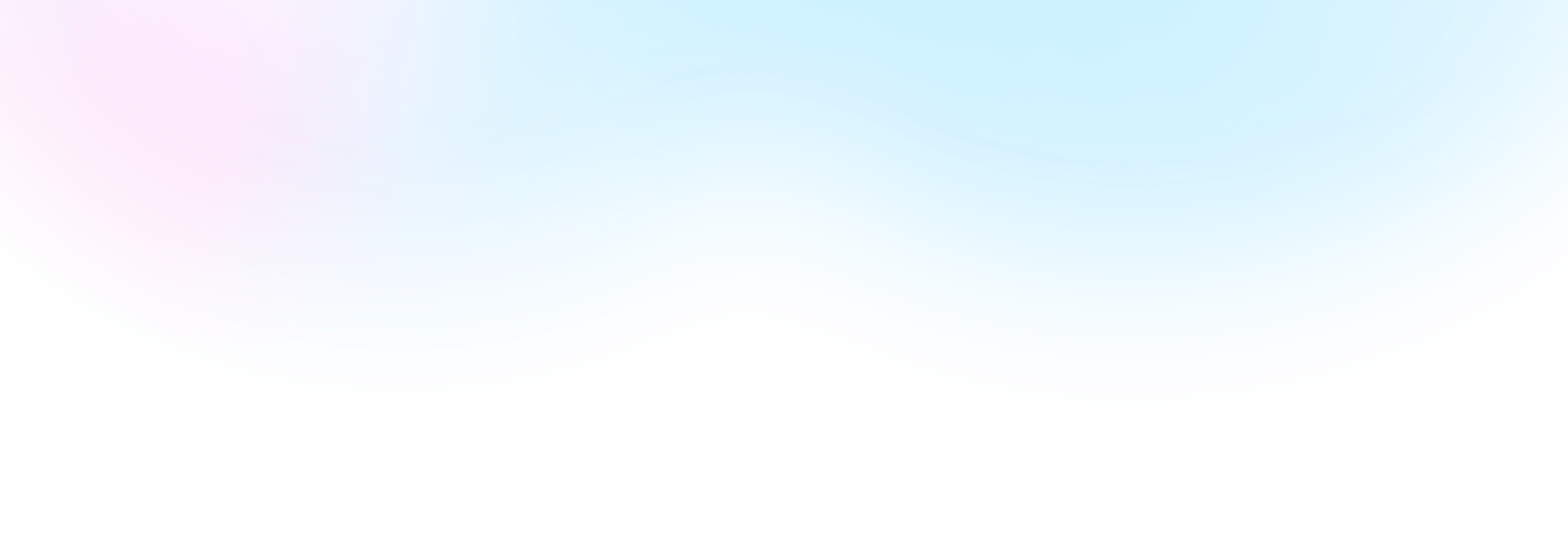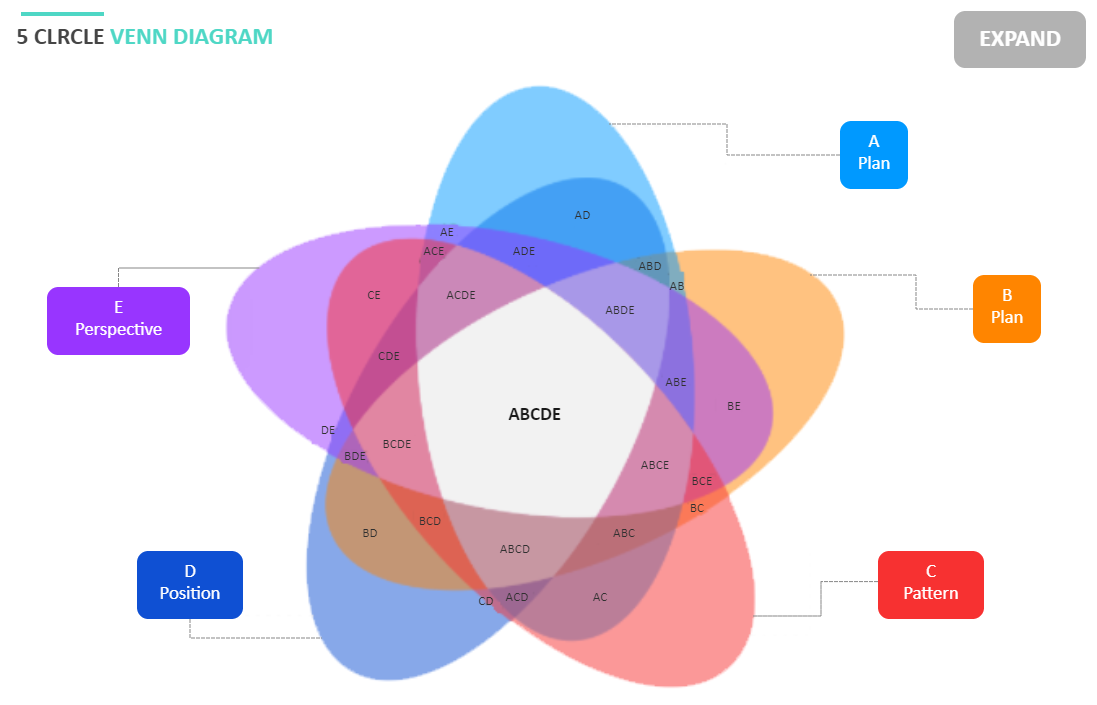Template Community / 3 Circle Venn Diagram

# 3 Circle Venn DiagramBlackwood
Published on 2022-04-20
Edit OnlineA three-circle Venn diagram, named after the English logician Robert Venn, is a diagram that uses three overlapping circles to show how the elements of three sets are related. When the three circles in a Venn diagram overlap, the overlapping parts share details with any two or all three circles. We can draw a three-circle Venn diagram to demonstrate the relationships between the factors 30, 40, and 100. Three circle Venn Diagrams are more complex than two circle diagrams. Venn diagrams can have multiple intersections and circle sets, but the three-circle or triple Venn diagram is the most commonly used.
Tag
venn diagram
Report
1
50PostRecommended Templates